# Darlington Transistor: What is it? (Darlington Pair)

Contents

## What is a Darlington Transistor?

A Darlington transistor (also known as a Darlington pair) is an electronics component made via the combination of two BJTs (Bipolar Junction Transistor) connected in such a way that it allows a very high amount of current gain. This is achieved through a compounding amplification, whereby the current is amplified by the first transistor and then further amplified by the second transistor.

As this compound structure is designed from two BJTs, this transistor is also known as “Darlington Pair”. This transistor behaves as a single unit transistor as it has only one emitter, collector, and base. The Darlington transistor was invented by Sidney Darlington in 1953.

If the current gain of a transistor is β1 and β2, the overall current gain of Darlington pair is β1β2. The current gain of this transistor is very high compared to the normal transistor. Therefore, this transistor is also known as “Super Beta Transistor”.

## Darlington Transistor Circuit

The Darlington Transistor consists of two PNP transistors or NPN transistors connected back to back. It is a single package with a common collector terminal for both transistors.

The Emitter terminal of the first transistor is connected with the base terminal of the second transistor. Hence, the base supply is given only to the first transistor, and the output current is taken only from the second transistor. Therefore, it consists of only one base, emitter, and collector as shown in the below figure.

There are two transistors Q1 and Q2.

Ib1 = Base current of transistor Q1
Ie1 = Emitter current of transistor Q1
Ib2 = Base current of transistor Q2
Ie2 = Emitter current of transistor Q2

In the above figure, two transistors are shown in one package. And from these two figures, the total base current (total input current) of the transistor package is equal to the base current of transistor Q1.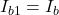Similarly, the total emitter current (total output current) of the package is equal to the emitter current of transistor Q2.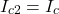Vbe1 = base-emitter voltage of transistor Q1
Vbe2 = base-emitter voltage of transistor Q2

Total base-emitter voltage is a summation of the base-emitter voltage of both transistors.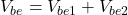β1 = Current gain of transistor Q1
β2 = Current gain of transistor Q2

The total current gain of the Darlington Transistor is βD. The total current gain of a transistor is the ratio of output current to the input current.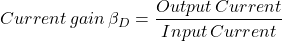(1)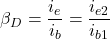In transistor, the Emitter current is summation of base current and collector current. And the collector current is β times of base current. Hence, in general form of transistor,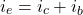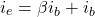(2)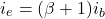For transistor Q2,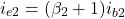From equation-(1),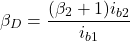From the circuit diagram, the emitter current of transistor Q1 is equal to the base current of transistor Q2.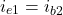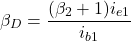For transistor Q1,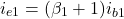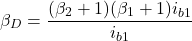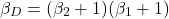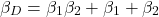In the above equation, the value of β1β2 is very large compared to the value of β1 + β2. Let’s take an example in which, the β1=100 and β2=100.

In this condition, β1β2 = 10000 and β12 = 200. Therefore, we can neglect the value of β12. And the gain of Darlington Transistor is,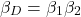## PNP and NPN Darlington Transistor

If the Darlington pair consists of both PNP transistors, it makes PNP Darlington Transistor. And if the Darlington pair consists of both NPN transistors, it makes NPN Darlington Transistor. The connection diagram of NPN and PNP Darlington Transistor is as shown in the below figure.

For both types of transistors, the Collector terminal is common. In the PNP transistor, the base current is given to the emitter terminal of the second transistor. And in NPN transistor, the emitter current is given to the base terminal of the second transistor.

The space required for the Darlington transistor is less compared to the space required for two transistors. Because here the collector terminal is common for both transistors.

## Darlington Transistor Switch

Let say we want to turn ON and OFF a load using a microcontroller. To do this task, first, we use an ordinary transistor as a switch, and second, we use a Darlington transistor. The circuit diagram of this configuration is as shown in the below figure.

In this condition, the current required for the load is 5A. And the microcontroller can draw only 20mA current to the base of the transistor.

Now, if we want to draw 5A current to the load, then,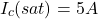The value of the current gain β of the normal transistor is about 100.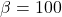And to drive this transistor in saturation mode, the value of base current is equal to at least,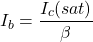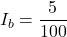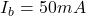To ensure the saturation condition and for deep saturation, the base current is 5 times of this value. Hence, the required value of the base current is 250mA.

But the microcontroller can draw only 20mA current to the base. Therefore, the load cannot turn ON by this microcontroller.

If we use the Darlington transistor as a switch, in this condition, the load current remains as it is. But the current gain of the Darlington transistor βd = 10000. Now, we calculate the required base current,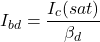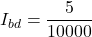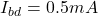And for deep saturation, we take 5 times base current of this value. Hence, the value of the base current in the case of the Darlington pair is 2.5mA. And this current is sufficient to draw by a microcontroller.

In this way, we can run the same load with the same microcontroller, if we use Darlington transistor as a switch.

## TIP120 Darlington Transistor

TIP120 is an NPN Darlington pair with the current gain is 1000. It is a good choice for interfacing a high current load with Arduino and microcontroller.

It has three terminals; pin-1 is the base terminal, pin-2 is collector terminal, and pin-3 is emitter terminal.

Pin-1 (Base) is used to turn ON and OFF transistor. The current flows through Pin-2 (Collector) is normally connected with the load. And the current flows through Pin-3 (Emitter) is normally connected to the ground.

The collector current of TIP120 is 5A. Hence, it can draw a load up to 5A. The peak value of the collector current is 8A. It is not designed to run continuously on 8A. It is just a peak current that can withstand by TIP120 for a short period.

The collector-emitter voltage (VCE) is 60V. If you need more voltage, you can use another transistor of TIP family, like TIP121 and TIP122 that can able to work on 80V and 100V respectively.

The equivalent circuit of TIP120 is as shown in the below figure.

## How to Test a Darlington Transistor?

To test the Darlington transistor is ON (working) or OFF (damaged) follow these steps.

Step-1: You need to identify, the base, collector, and emitter terminals. And you must know it is PNP or NPN transistor. These steps refer to the NPN Darlington pair. The test leads are reversed for the PNP transistor.

Step-2: Set the dial of a digital multimeter (DMM) on the diode symbol. If it is not available in multimeter, set the multimeter on low resistance mode.

Step-3: Connect the positive lead of multimeter with a base terminal of the transistor and connect the negative lead of multimeter with collector and then emitter.
If the transistor is functioning well, the multimeter shows low hFE reading (transistor current gain).

Step-4: Now, reverse the leads and connect the positive lead with collector and emitter terminals. Connect the negative lead with the base terminal.
Due to the reverse bias test, it displays an open circuit or infinite resistance.

A Darlington transistor (i.e. a Darlington pair) has several advantages compared to the normal transistor. They have been summarized in the list below:

• The main advantage of a Darlington transistor is the high current gain. So, a small amount of base current can trigger the transistor.
• It offers high input impedance which translates into an equal decrease in output impedance.
• It is a single package. So, it is easy to configure on a circuit board or PCB compared to connect two different transistors.

The disadvantages of a Darlington transistor (i.e. a Darlington pair) have been summarized in the list below:

• It has a slow switching speed.
• The base-emitter voltage is almost two times compared to a normal transistor.
• Due to high saturation voltage, in such an application, it dissipates high power.
• The bandwidth is limited.
• The Darlington transistor introduces a phase shift at a certain frequency in the negative feedback circuit.

Want To Learn Faster? 🎓
Get electrical articles delivered to your inbox every week.
No credit card required—it’s 100% free.